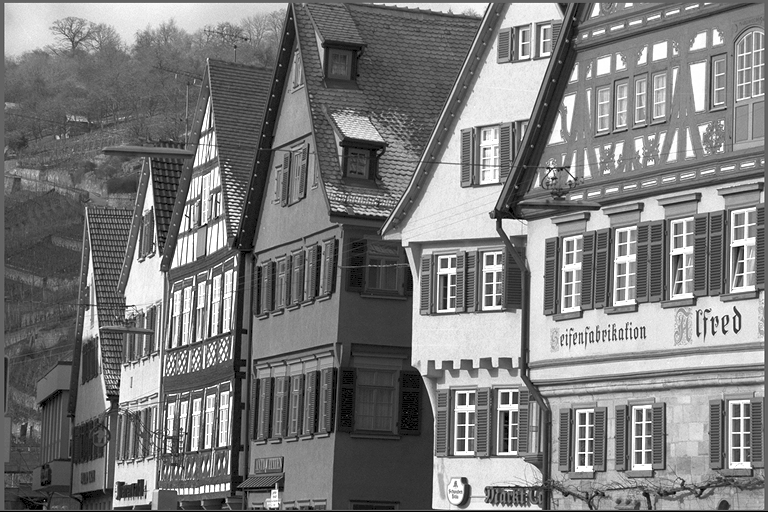Gaussian Filter

# Overview

Gaussian Filter is a low-pass discrete Gaussian filter that smooths out the image by doing a Gaussian-weighted averaging of neighbor pixels of a given input pixel. It produces images with less artifacts than Box Filter, but could potentially be more costly to compute.

It supports two modes of operation:

• Kernel support size is automatically calculated based on the filter standard deviation (sigma).
• Use both user-provided kernel support size and filter standard deviation.
Input Gaussian kernel Output7x7 support,

$\sigma=1.7$# Implementation

Gaussian filter is implemented as a convolution operation on the input image where the kernel has the following weights:

$w_g[x,y] = \frac{1}{2\pi\sigma^2} \cdot e^{-\frac{x^2+y^2}{2\sigma^2}}$

When the input kernel support size is 0 for a given dimension (or both), it is calculated from the given standard deviation by assuming that the weights outside $$\pm3\sigma$$ window are zero.

In this case, the following formula is used:

$w = \max\{3,2 \times \lceil 3\sigma\rceil-1\}$

Note
We clamp the minimum kernel size to 3 because a kernel with size 1 doesn't have enough samples to properly characterize a Gaussian function.

# C API functions

For list of limitations, constraints and backends that implements the algorithm, consult reference documentation of the following functions:

Function Description
vpiSubmitGaussianFilter Runs a 2D Gaussian filter over an image.

# Usage

Language:
1. Import VPI module
import vpi
2. Use the CPU backend to filter the input image with a 7x7 Gaussian kernel with $$\sigma=1.7$$, using ZERO boundary condition. Input and output are VPI images.
with vpi.Backend.CPU:
output = input.gaussian_filter(7, 1.7, border=vpi.Border.ZERO)
1. Initialization phase
1. Include the header that defines the Gaussian filter function.
Declares functions that implement the Gaussian Filter algorithm.
2. Define the input image object.
VPIImage input = /*...*/;
struct VPIImageImpl * VPIImage
A handle to an image.
Definition: Types.h:256
3. Create the output image. It gets its dimensions and format from the input image.
int32_t w, h;
vpiImageGetSize(input, &w, &h);
vpiImageGetFormat(input, &type);
VPIImage output;
vpiImageCreate(w, h, type, 0, &output);
uint64_t VPIImageFormat
Pre-defined image formats.
Definition: ImageFormat.h:94
VPIStatus vpiImageGetFormat(VPIImage img, VPIImageFormat *format)
Get the image format.
VPIStatus vpiImageCreate(int32_t width, int32_t height, VPIImageFormat fmt, uint64_t flags, VPIImage *img)
Create an empty image instance with the specified flags.
VPIStatus vpiImageGetSize(VPIImage img, int32_t *width, int32_t *height)
Get the image dimensions in pixels.
4. Create the stream where the algorithm will be submitted for execution.
VPIStream stream;
vpiStreamCreate(0, &stream);
struct VPIStreamImpl * VPIStream
A handle to a stream.
Definition: Types.h:250
VPIStatus vpiStreamCreate(uint64_t flags, VPIStream *stream)
Create a stream instance.
2. Processing phase
1. Submit the algorithm to the stream along with other parameters. It'll be executed by the CPU backend. It defines a Gaussian filter with 7x7 support and $$\sigma=1.7$$ in both horizontal and vertical directions, along with a zero border extension.
vpiSubmitGaussianFilter(stream, VPI_BACKEND_CPU, input, output, 7, 7, 1.7, 1.7, VPI_BORDER_ZERO);
VPIStatus vpiSubmitGaussianFilter(VPIStream stream, uint64_t backend, VPIImage input, VPIImage output, int32_t kernelSizeX, int32_t kernelSizeY, float sigmaX, float sigmaY, VPIBorderExtension border)
Runs a 2D Gaussian filter over an image.
@ VPI_BACKEND_CPU
CPU backend.
Definition: Types.h:92
@ VPI_BORDER_ZERO
All pixels outside the image are considered to be zero.
Definition: Types.h:278
2. Optionally, wait until the processing is done.
vpiStreamSync(stream);
VPIStatus vpiStreamSync(VPIStream stream)
Blocks the calling thread until all submitted commands in this stream queue are done (queue is empty)...
3. Cleanup phase
1. Free resources held by the stream and the input and output images.
vpiImageDestroy(output);
void vpiImageDestroy(VPIImage img)
Destroy an image instance.
void vpiStreamDestroy(VPIStream stream)
Destroy a stream instance and deallocate all HW resources.

For more information, see Gaussian Filter in the "C API Reference" section of VPI - Vision Programming Interface.

# Performance

For information on how to use the performance table below, see Algorithm Performance Tables.
Before comparing measurements, consult Comparing Algorithm Elapsed Times.
For further information on how performance was benchmarked, see Performance Benchmark.

-